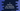# C program to find the first non repeating character in a string### C program to find the first non repeating character in a string :

In this tutorial, we will learn how to find the first non-repeating character in a string using C. For example, for the string codevscolor, it will print d as it is the first non-repeating character. We can solve this problem different ways. Let’s take a look at them one by one :

### Approach 1 : O(n^2) :

1. Use two loops, one inside another.
2. The outer loop will read the string character by character. The inner loop will check each character by scanning each characters of the string if it is repeating or not.
3. This approach is O(n^2) complexity. If our string is of million characters, then it will take a huge amount of time.

### Approach 2 : O(n) :

1. We will use one HashMap. HashMap map one key to one value. We will use one integer array as a hashmap.
2. The key of the hashmap is the ASCII value of a character and the value is its total number of count in the string.
3. Since, total 256 ASCII values are available, this hashmap will be of size 256.
4. Using one loop, we will scan each character of the string one by one and for each character , we will increment the value of the hashmap for that key position. For example, for codevscolor, first character is c. So, we will increment the value of hashmap[‘c’]. The ASCII value of c is 99 . So the value of hashmap will become 1. Similarly, we will do this for all other characters.
5. After all characters are scanned, the program will read the same string again and for each character it will compare the value in the hashmap. If it is 1, means that character occurs only one time in the string, it will print that character.
6. So, we have traverse the string two times in this process. Hence the time complexity is O(n).

### Approach 3 : O(n) : Modified version of the above method :

In the above process, we are scanning the string two times. This method is good but for a large string, eg for a string of size 1 million, we will have to scan 1 + 1 = 2 million characters. This method can be improved by storing two values in the hashmap : index and total count. This way, for the second iteration, we will iterate over the hashmap to find out the first non repeating character i.e. we will iterate only 256 characters on second time.

We have explain the third method below. Let me know if you have any queries :

### C program :

``````#include
#include
#include
#define MAX_SIZE 256

//5
struct element{
int count;
int index;
};

//6
int findFirstNonRepeatedChar(char *str){
//7
struct element *recordTable = (struct element *)calloc(sizeof(struct element),MAX_SIZE);
//8
int i;
int resultElementIndex = -1;

//9
for(i=0 ; *(str+i) ; i++){
if(!isspace(*(str+i))){
recordTable[*(str+i)].count ++;
recordTable[*(str+i)].index = i;
}
}

//10
for(i=0; i < MAX_SIZE ; i++){
//11
if(recordTable[i].count == 1){
//12
if(resultElementIndex == -1){
resultElementIndex = recordTable[i].index;
}else{
if(recordTable[i].index < resultElementIndex){
resultElementIndex = recordTable[i].index;
}
}
}
}

//13
return resultElementIndex;
}

int main(){
//1
char str;

//2
printf("Enter a string : \n");
fgets(str,100,stdin);

//3
int index = findFirstNonRepeatedChar(str);

//4
if(index == -1){
printf("No element found \n");
}else{
printf("First non-repeated character %c \n",str[index]);
}
}``````

### Explanation :

The commented numbers in the above program denote the step-number below :

1. Create one char array to store the user-input string.
2. Ask the user to enter a string and store it in str variable.
3. Get the index of the first non-repeating character by calling function findFirstNonRepeatedChar. It returns the index of the character and -1 if no element is found.
4. Print the character if found.
5. structure element consists of two integers count and index to store the total count and index of a character.
6. findFirstNonRepeatedChar function takes one char-array as input and returns the first non-repeating char index. If no char found, it returns -1
7. Create one hashMap recordTable. It can hold elements of type ‘element structure’. Its length is 256.
8. Create one integer i to use in the loop and one element resultElementIndex to store the index of first non-repeated char.
9. Run one for-loop to read each character of the string. Increment the count and change the index for that position of the hashmap. e.g., for character ‘c’, we will change the values for resultElementIndex[‘c’] or resultElementIndex. We are checking only non-empty characters here.
10. Now, run one more for-loop to read elements of the hashmap.
11. If the count is 1 for an element, check it.
12. If current stored index is -1 set the index of this element. Otherwise , check if this element’s index is less than stored index or not. If yes, set this index as result index.
13. Return the stored result index.

### Sample Output :

``````Enter a string :
codevscolor
First non-repeated character d

Enter a string :
Hello World
First non-repeated character H

Enter a string :
aa bb cc
No element found``````